# Heat capacity vs Thermal conductivity in a LN2 bath

• tempneff

#### tempneff

I worked on a lab experiment that was meant to measure heat capacity but left me with some other questions. The students measured the mass of a cup of liquid nitrogen as it boiled off, recording mass vs time. Then they drop a solid object into the bath, one experiment with a small bit iron bar, then with a piece of quartz in a separate run. The boil off rate increases for some time then returns to the first rate once the system equilibrates. Given the latent heat of vaporization of LN2, they then successfully calculated the Specific Heat of the iron C = ~450 J/kg°C and quartz C = ~730 J/kg°C.

My question is in regard to the rate at which the objects cooled assuming when we measured grams/ per second of LN2 that is analogous to the rate of cooling. The mass of these objects were roughly the same, so I expected the iron to cool fastest since it has a higher thermal conductivity, but the quartz was significantly faster every time. I can guess at a few things. Maybe it was geometry? the iron bar was smooth and the quartz was porous and roughly shaped with more surface area of contact? or maybe the iron did cool faster but the thermal conductivity decreases as it cools. maybe the rate was driven by the LN2 and the stored heat, not the transport propertied of the materials.

What drives the rate in this scenario?

To a first approximation, it is the thermal diffusivity.

•tempneff
Sure, but the thermal diffusivity of iron is an order of magnitude greater than quartz. This is why it doesn't make sense to me. I think there must be some dependence on the energy available. Below is a plot of the data that has been normalized. I thought maybe I'd try and match the amount of heat expected in the samples by tweaking the mass. I use ratio of Specific Heat Capacity of the two material 450/730 = .616. Then I took the mass of iron was 44.62 and determined that I should use ~27.6 gs of quartz. If we look at the red lines vs black with a square, these slopes begin to line up. The larger the mass of quartz the steeper rate of transfer (diamond and circle in black)

If it was strictly driven by the transport properties, and I am right about matching the available energy, shouldn't the metal have a steeper line?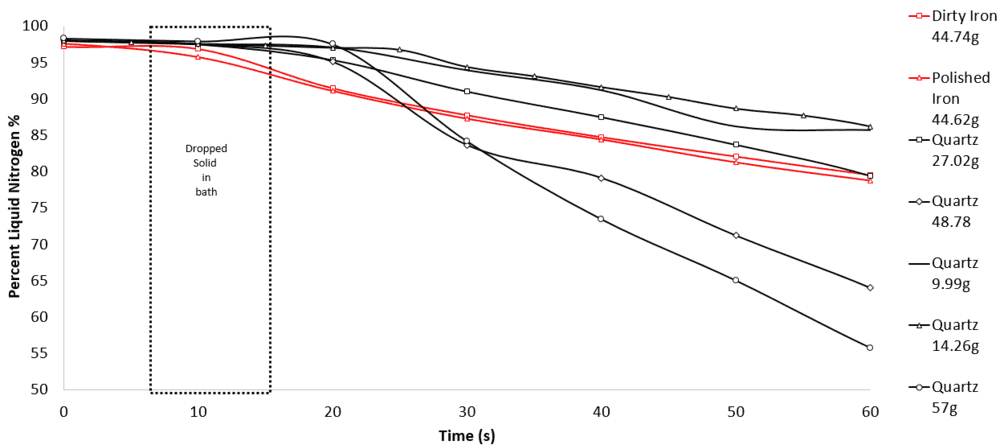The thermal diffusivity is, of course, not the only parameter involved. The surface area of the sample and its volume and shape are also going to be important. Do you know how to model this?

Suppose you had spherical samples, for example, and their surfaces were put into contact with a colder specified temperature, would you be able to estimate the total amount of heat transferred out of the sample? Would you be able to model this?

•tempneff
I'm an EE in charge of the instruments for a university physics dept. I make sure their labs work, but I leave the modeling for the students. In this case I am interested because the data was counter intuitive to me.

I'm an EE in charge of the instruments for a university physics dept. I make sure their labs work, but I leave the modeling for the students. In this case I am interested because the data was counter intuitive to me.
Fair enough. OK. To help you with this, I first need to do some scouting calculations to get the lay of the land. Please choose one representative sample of each materials and provide me with the shape/dimensions of the sample. Then, rather than the percent liquid nitrogen, please provide the actual weight of the liquid nitrogen as a function of time (unless the amount is always the same). In each case, please specify the starting amount of liquid nitrogen and its temperature. Is the liquid nitrogen at its boiling point (-196C), or is it below?

•tempneff
Wow, thanks for helping! I'm attaching a spreadsheet and I think it has everything you need. The quartz samples have approximations on dimensions because they are raw chunks, not single crystal. Measuring their surface area is certainly impractical without sophisticated equipment. Please let me know if anything is unclear.

#### Attachments

• SpecificHeatLab_RawData.xlsx
16.1 KB · Views: 205
Wow, thanks for helping! I'm attaching a spreadsheet and I think it has everything you need. The quartz samples have approximations on dimensions because they are raw chunks, not single crystal. Measuring their surface area is certainly impractical without sophisticated equipment. Please let me know if anything is unclear.
Question: For the quartz, the dimensions and masses do not seem to be consistent with the known density of quartz 2.66 gm/cc. They seem more consistent with a density of 1.6. Comments?

•tempneff
Several thoughts. Foremost, my measurements approximate the rock into a rectangular cross section which is a big assumption. I did take some care in this estimation but there is a lot of room for error. Second, these are loose grained rocks, I suppose the measured density must depend also on the porosity or the mineral and the percentage of single crystal quartz. Third, maybe it's not quartz. This is an old department and no one knows where the bag of "quartz" came from.

Although exact geometry is not easily measured, thanks to Archimedes the density is. Would it help if I got a real number for density?

Last edited:
Several thoughts. Foremost, my measurements approximate the rock into a rectangular cross section which is a big assumption. I did take some care in this estimation but there is a lot of room for error. Second, these are loose grained rocks, I suppose the measured density must depend also on the porosity or the mineral and the percentage of single crystal quartz. Third, maybe it's not quartz. This is an old department and no one knows where the bag of "quartz" came from.

Although exact geometry is not easily measured, thanks to Archimedes the density is. Would it help if I got a real number for density?
Yes. I should also mention that, based on my scouting calculations, a picture is emerging. But I want to hold off a little longer on discussing this.

•tempneff
Make a plot of ##\frac{\Delta N2}{MC}## versus time for all of the samples, where ##\Delta N2## is the absolute amount of nitrogen that have evaporated up to time t, M is the mass of the sample, and C is the sample heat capacity. What do you obtain?

•tempneff
I get a plot that looks like this (if I did it right). I'm not sure what to make of it though. Kinda looks like some the quartz samples were not completely cooled by the time I stopped taking data. What am I missing?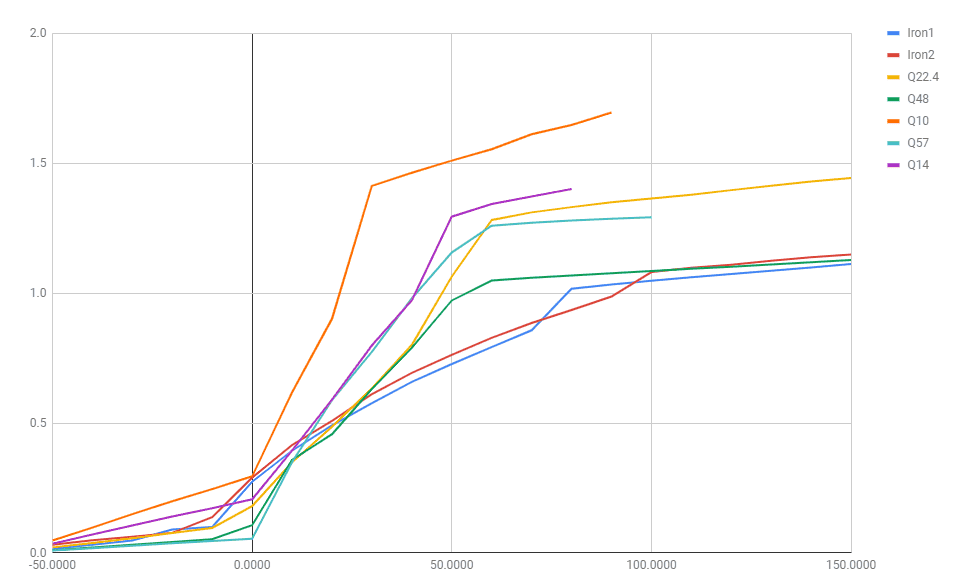I get a plot that looks like this (if I did it right). I'm not sure what to make of it though. Kinda looks like some the quartz samples were not completely cooled by the time I stopped taking data. What am I missing?
View attachment 245206
This plot tells us several things. First of all, it looks either like (a) none of the cases reached steady state or (b) there was nitrogen evaporation occurring (at roughly a constant rate) even before and after the samples reached steady state. I think the latter is most likely. So we should subtract the background evaporation rate times time divided by MC from each of the curves. The background evaporation rate should be the same for all the cases. From this data, what do you estimate that to be?

Secondly, the final amount of N2 that is lost as a result of heat exchange with the samples should be given by: $$\Delta N2_{final}H=MC(25-(196))$$where H is the heat of vaporization of N2 (200 J/gm). If we rearrange this equation, we obtain: $$\frac{\Delta N2_{final}}{MC}=\frac{(25-(-196))}{H}=1.1$$ Therefore, all the curves should asymptote to a value of 1.1

Last edited:
•tempneff
We certainly do have background evaporation. The lab intends to observe the background for some time, then add the solid, then wait until the system returns to just the background. I have ~.033 g/s for background evaporation, from the data.

So we should subtract the background evaporation rate times time divided by MC from each of the curves.

Why are we including MC in the background correction?

We certainly do have background evaporation. The lab intends to observe the background for some time, then add the solid, then wait until the system returns to just the background. I have ~.033 g/s for background evaporation, from the data.

Why are we including MC in the background correction?
I confirm the 0.033 g/s. We are including the MC in the background correction because it is in the total loss parameter, ##\Delta N2/(MC)##. Please show how the graph changes when we include this correction.

•tempneff
So does that just become a plot of ##\frac{\Delta N2-0.033}{MC}## versus time?

So does that just become a plot of ##\frac{\Delta N2-0.033}{MC}## versus time?
No. ##\frac{\Delta N2-0.033t}{MC}## vs time. There is a factor of t.

I've been looking at the values of thermal diffusivity for iron and quartz. They are 0.28 cm^2/sec and 0.0051 cm^2/sec respectively. For a 2 cm diameter iron sphere with these values, we find that it would take about seconds for an iron sphere to equilibrate with a new surface temperature, if its surface temperature had been suddenly changed to a new value and then held at that value. For a quartz sphere of the same diameter, the corresponding time would be about 3 minutes. Since the time scale for the nitrogen vaporization to take place in these experiments is much larger than this, we must conclude that the main resistance to heat transfer in the experiments resided in the nitrogen vapor layers surrounding the various samples. This means that the nitrogen evaporation time in the experiments did not depend significantly on the thermal conductivities of the iron and quartz. The results would have been the same if they were infinite.

•tempneff
Here is the plot after correction. I think your assessment makes sense. Do you think that the dependence of boiling regimes (specifically Transition Boiling) on surface area and roughness could explain why the rough quartz is less restricted. Do we agree that, from the data, there is a trend in the size of the samples with the transfer rate? Maybe then the larger masses simply provide a greater surface area for transfer?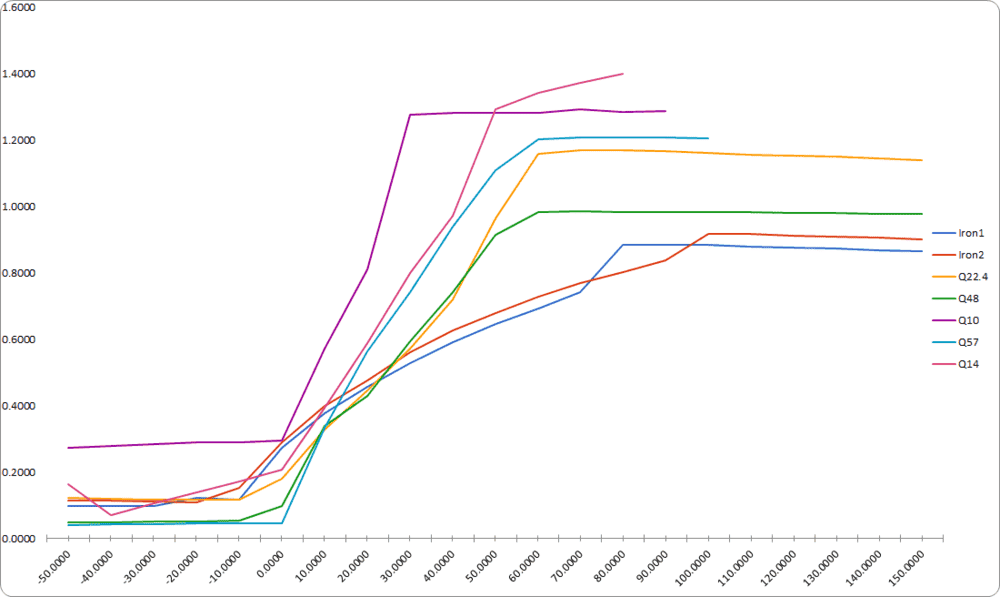Here is the plot after correction. I think your assessment makes sense. Do you think that the dependence of boiling regimes (specifically Transition Boiling) on surface area and roughness could explain why the rough quartz is less restricted. Do we agree that, from the data, there is a trend in the size of the samples with the transfer rate? Maybe then the larger masses simply provide a greater surface area for transfer?
View attachment 245291
Nicely plotted.

But, whoa pardner. Let's take a step backwards and slow down.

Before we address the time response, we need to get a better understanding of why the results don't all asymptote to 1.1. The iron seems to be asymptoting to 0.9 and the quartz seems to be asymptoting to about 1.2. The quartz results could be improved by lowering each of the curves by the values shown at time t = -10 min, to remove the initial offset that was there before the sample was even inserted. Please do that next. I think it will result in all the quartz curves better asymptoting to 1.1 (the expected value). However, it will also lower the iron curves a little more. Let's see what we get when you do this.

Meanwhile, I am going to do a heat transfer analysis to analyze the problem mathematically. This will enable us to evaluate the transient behavior better (and will tell us how to next properly scale the time parameter).

•tempneff and BvU
The porosity of the quartz samples is only about 50-60%, so the ratio of their surface areas to their masses is higher than the iron. Please calculate the surface area to mass ratios of the various samples, including the iron, assuming that the quartz samples have the same rectangular parallelepiped shapes that you have listed. Then calculate the ratio of surface area to MC for each sample. This will give us an idea how to scale the curves in the time direction. Thanks.

•tempneff
Incidentally, the temperature change of the samples is 220 C, not the 170 C indicated on the spreadsheet.

•tempneff
Yes, I corrected that delta T error. Here is the shifted plot you asked for.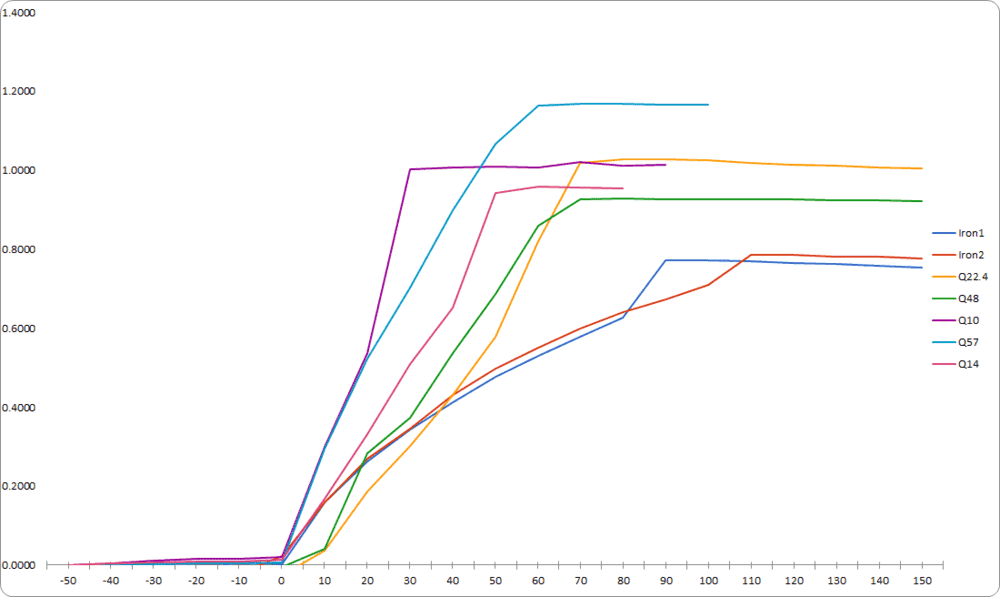Shouldn't Quartz #1 be 27.5 gm, rather than 22.4 gm? And what happened to Quartz # 2 with 52 gm?

•tempneff
I think a picture is emerging now.

The heat transfer analysis on the samples goes like this. The transient heat balance on a sample is given by
$$MC\frac{dT}{dt}=-hS(T-T_N)$$ where S is the surface area of the sample, ##T_N## is the nitrogen temperature, and h is the heat transfer coefficient between the sample and the nitrogen. In this analysis, we will assume that the heat transfer is dominated by the resistance on the nitrogen side of the boundary and that h is roughly constant in time and independent of the type of sample. The solution to this equation, subject to the initial temperature condition of the sample is:
$$T=T_N+(T_0-T_N)e^{-\frac{hSt}{MC}}$$where ##T_0## is the initial sample temperature. From this it follows that the amount of heat given up by the sample up to time t is given by:
$$Q=MC(T_0-T_N)(1-e^{-\frac{hSt}{MC}})$$ This must be equal to the amount of nitrogen lost up to time t time the heat of vaporization H:
$$\Delta N_2H=MC(T_0-T_N)(1-e^{-\frac{hSt}{MC}})$$or, rearranging,
$$\frac{\Delta N_2}{MC}=\frac{(T_0-T_N)}{H}(1-e^{-\frac{hSt}{MC}})$$
This is basically the relationship we have plotted on our graph, aside from the scale factor ##\frac{hS}{MC}## on the time.

OK so far??

•tempneff
Catching up..
Yes the number from Quartz #1 was wrong, an accident. Quartz #2 is back, also an accident. A new plot and a table of ratios are both below.
OK so far??
Yes, it makes sense. Is it fair to say that the resistance on the nitrogen side of the boundary functionally determines the rate of transfer.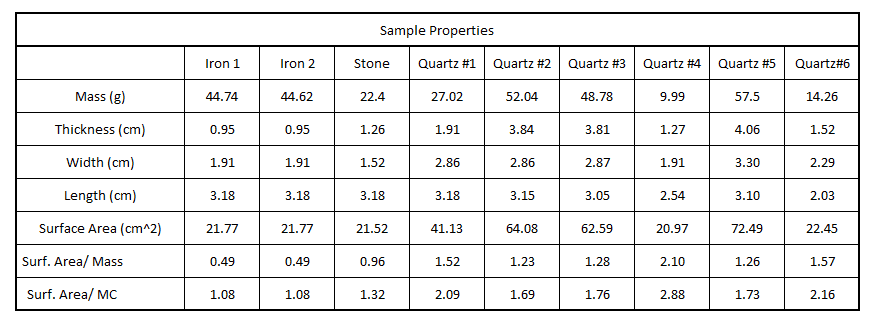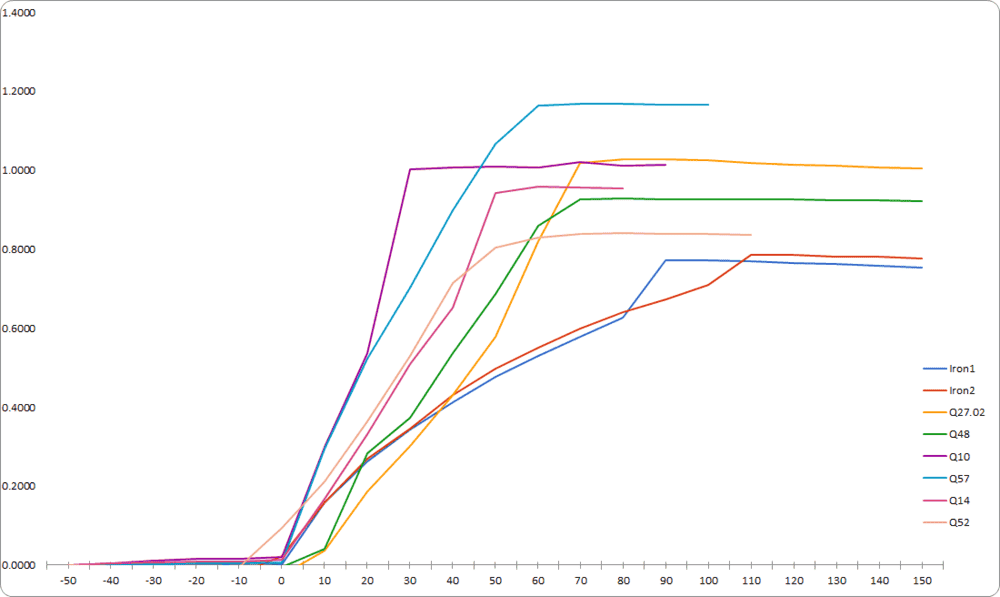Yes, it is correct to say that the resistance on the nitrogen side of the boundary (together with the surface area S and MC) functionally determines the rate of heat transfer.

The calculated values of the Surf. area/MC are extremely revealing. In the equation we wrote, since the heat transfer coefficient h is assumed constant, the higher the value of S/MC, the more rapid the rise in ##\Delta N_2/MC## should be. And the values of this parameter for the quartz samples are much higher than those of the iron samples. Therefore, this is the reason they rise much more rapidly.

S/MC is essentially a scaling parameter on the time. If we multiply the time by this parameter for all the samples, theoretically, it should cause all the curves to superimpose onto a single curve when we replot the graph using the scaled time as the abscissa. Of course, in the case of the quartz samples, the surface area is highly uncertain due to the very irregular shape, and the values of the area obtained assuming perfect rectangular parallelepipeds is probably not a good approximation to the actual surface area. Still, if we multiply the times for all the quartz samples by a factor of about 2, it would put the quartz curves pretty close to the iron curves.

So, I believe that we have identified the correct mechanistic explanation of the observed behavior. Maybe, if you feel like it, you might try scaling the times for the samples by their individual S/MC values and redoing the plot just to see. But I think that, at this point, it would not add much to better quantify the comparison.

•tempneff
Well I'll be darned... Superposition is a lot to ask in the real world, but I say this is getting close. Thanks for working through this with me, now I can sleep at night.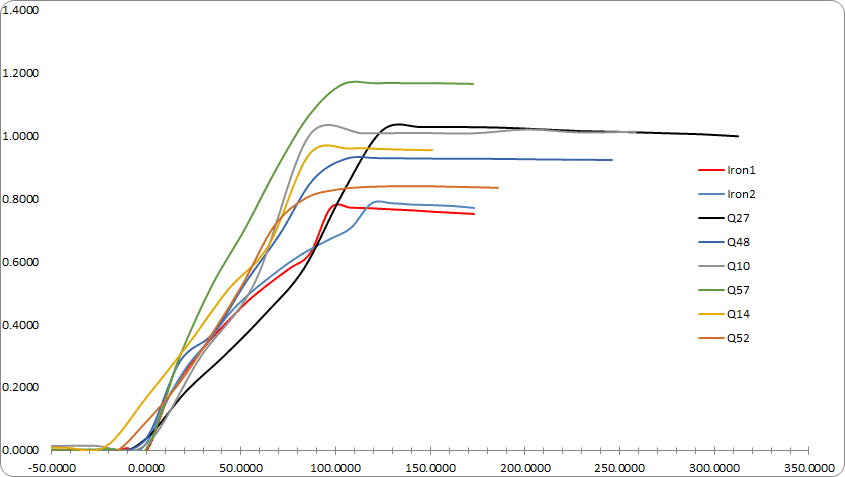•Chestermiller